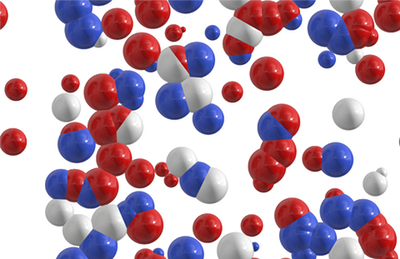### Moles and the Mole ConceptIn chemistry, a mole (mol) is a unit of measure that measures the quantity of something. Science requires that all units of measure be based on something that can be theoretically reproduced. A mole, scientifically speaking, is the quantity of a substance that has the same number of particles as is found in 12.000 grams of carbon-12. In numerical form, a mole is approximately 6.023 x 1023. This number is called Avogadro's Number.

Why Bother with Moles?

Avogadro's Number is huge, and many beginning chemistry students wonder just why they need to bother with it in the first place. When chemical reactions occur, measuring the particles using grams simply would not work because of the minute nature of the atoms or molecules or ions. However, in many reactions, measuring the exact number of these substances isn't practical either. So scientists came up with the mole concept to give them a way to measure the quantity of molecules or ions in a reaction.

The mole concept seems confusing because it is such a large number. However, in practical terms, a mole equals 6.023 x 1023 "things," whether they be atoms, molecules, ions, or particles. This is no different than saying one dozen equals 12 "things." Working with 1 mole is much easier than working with 6.023 x 1023 particles when performing chemistry equations, which is why chemists created the mole concept.

The Role of the Mole Concept in Chemistry

A mole is a number of particles, so a mole of one substance can have a different mass than a mole of another substance, just as a dozen feathers will have a different mass than a dozen bricks. The mass of one mole of an atom is equal to the atomic mass of the element. So a mole of sodium, which has an atomic mass of 23, has a mass of 23 grams. Because of this, the mole concept makes it possible for chemists to convert from number of particles to mass in their equations.

### Subscribe Now!

Subscribe to our Free Experiment of the Month Club

*
*
*